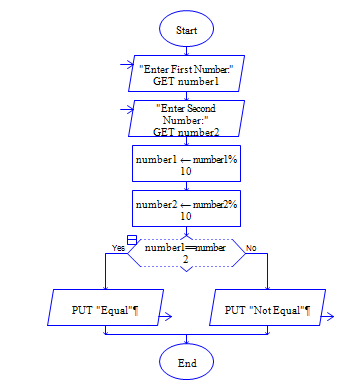# Java Program to find the whether the last digit of given two numbers are equal or not

Get two numbers num1 and num2 and check whether last digit of num1 and num2 are equal or not?.

Sample Input 1:

65 45

Sample Output 1:

Last Digits are Equal.

Sample Input 2:

35 38

Sample Output 2:

Last Digits are Not Equal

#### Flow Chart Design#### Program or Solution

``` import java.util.*; class LastDEqual { public static void main(String args[]) { int num1,num2; Scanner sc=new Scanner(System.in); System.out.println("Enter two numbers:"); num1=sc.nextInt(); num2=sc.nextInt(); if(num1%10==num2%10) { System.out.println("Last Digits Are Equal."); } else { System.out.println("Last Digits Are Not Equal."); } } } ```

#### Program Explanation

1. Get two inputs num1 and num2

2. extract the last digits of num1 and num2 using %10,

3. then compare last digits.